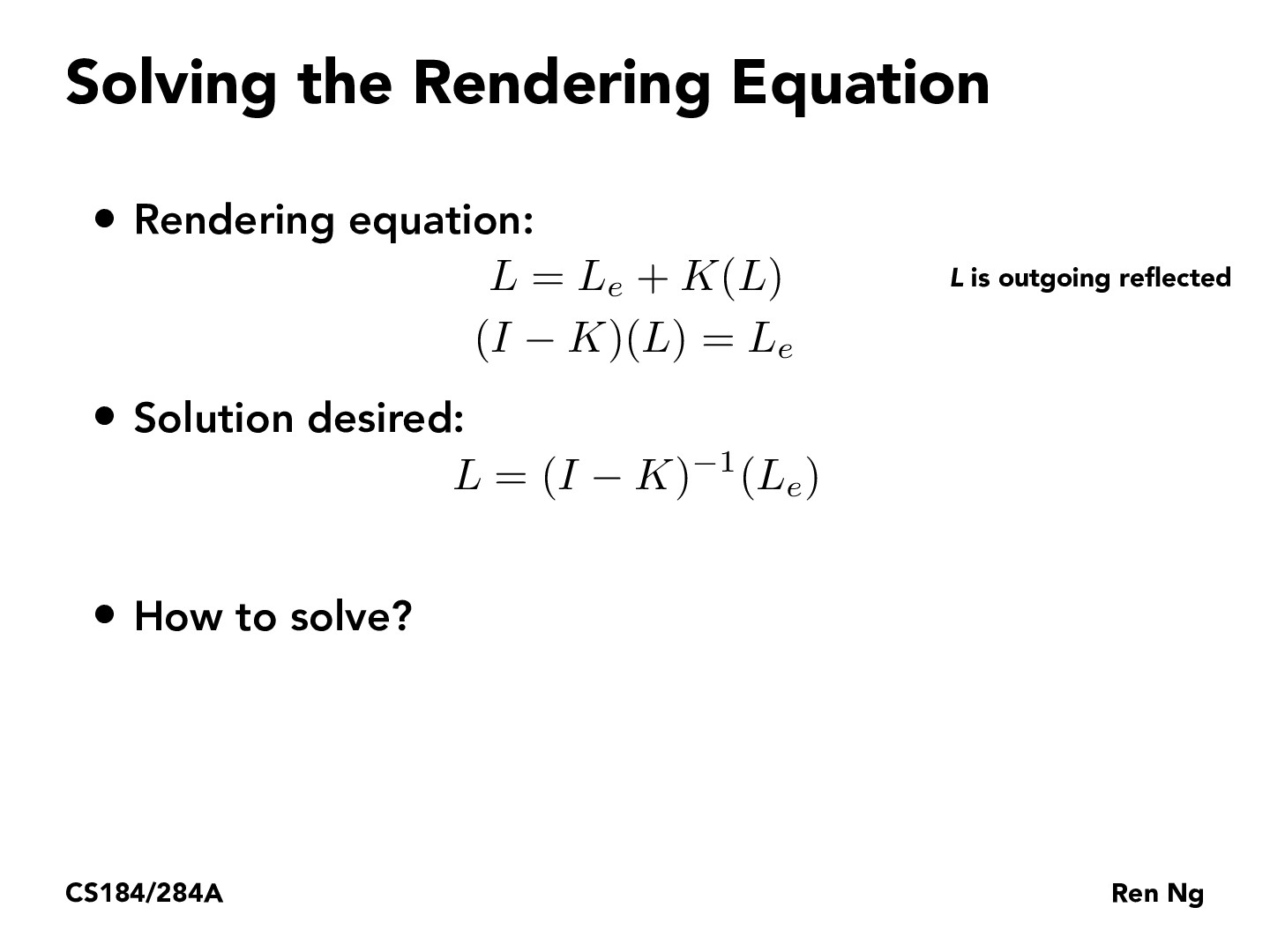You are viewing the course site for a past offering of this course. The current offering may be found here.Lecture 13: Global Illumination & Path Tracing (39)ChrisP19

This setup reminds me of solving the Bellman equations in reinforcement learning. The Bellman equations are also a set of recursive relationships that can be written using operator notation. However, they are usually solved iteratively via a dynamic programming approach, so I wanted to see if we could also apply that approach to the simpler case here. Let's say the variable $L_i$ represents the light after i bounces/steps. Then:

Initialize $L_0 = 0$
$L_1 = L_e + K(L_0) = L_e$
$L_2 = L_e + K(L_1) = L_e + KL_e$
$L_3 = L_e + K(L_2) = L_e + KL_e + K^2L_e$

So it does work- as we keep computing more steps, this solution will match the solution given in the next slide.

You must be enrolled in the course to comment